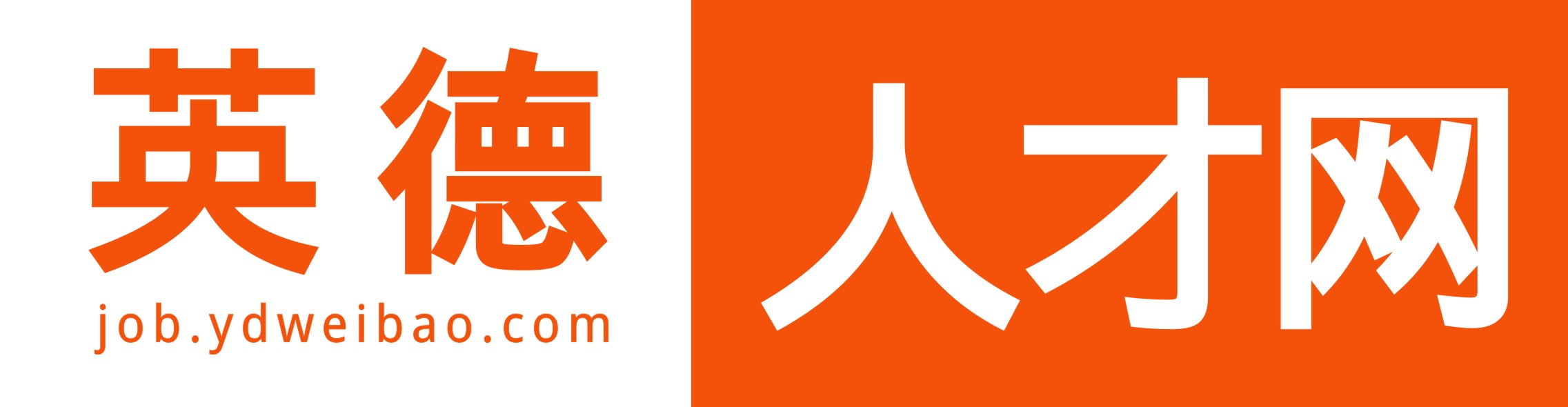• 登录 | 注册• 不限
• 市场销售
• 行政人事
• 城建装修
• 客户服务
• 会计财务
• 普工技工
• 文体培训
• 文职文员
• 设计包装
• 百货零售
• 工厂工业
• 餐饮休闲
• 金融保险
• 管理运营
• 物流贸易
• 司机后勤
• 网络硬件
• 机械仪表
• 咨询顾问
• 电子通讯
• 医疗美容
• 翻译法律
• 轻工工艺
• 化工制药
• 摄影影视
• 能源环保
• 编辑发行
• 其他分类

• 不限
• 财务主管/经理
• 会计
• 会计助理
• 注册会计师
• 注册审计师
• 审计
• 总账主任
• 出纳
• 审计(核)主管
• 统计
• 财务分析
• 成本分析/核算
• 帐目(进出口)管理
• 营运会计经理
• 审计会计经理
• 会计财务其他相关职位

• 英城镇
• 英红镇
• 东华镇
• 望埠镇
• 石灰铺镇
• 沙口镇
• 大站镇
• 九龙镇
• 浛洸镇
• 连江口镇
• 白沙镇
• 桥头镇
• 青塘镇
• 黎溪镇
• 水边镇
• 西牛镇
• 九龙镇
• 大湾镇
• 大洞镇
• 黄花镇
• 横石水镇
• 石牯塘镇
• 横石塘镇
• 下太镇
• 清远市
• 广州市
• 佛山市

• 不限
• 今天
• 三天内
• 一周内
• 一个月内
• 三个月内

• 不限
• 全职
• 兼职

• 不限
• 1000元以下
• 1000-2000元
• 2000-3000元
• 3000-5000元
• 5000-8000元
• 8000-1.2万元
• 1.2万-2万元
• 2万元以上
• 面议